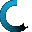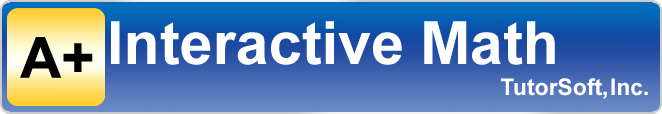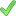Retrieving data ...
Please do not click anywhere else.

If you see no response after 2-3 minutes, then click on the [X] button in the upper right corner to close the window and retry the operation at a later time.
Interactive Multimedia Learning & Teaching Management System Suite• Q1.  What is NEW "Adaptive Placement Testw/ Lesson Plan" from A+ interactive Math?

It is a comprehensive program to test student's skill level, identify learning gaps (if any) and close the learning gaps with everything you need to close the learning gaps. It is easy to use, intuitive and includes comprehensive placement testing, fun lessons with interactive review and plenty of practice problems with step-by-step instructions to ensure your students master various essential math concepts. It helps you "Build a strong foundation in math you can count on"!
And all this for just \$29.99 - the BEST  \$29.99 you would spend on your child's math education!

• Q2.  Do I have to use your curriculum to take the placement test and/or after closing the gaps with this program?

No. You can use any math curriculum that is out there. Each math curriculum should be teaching certain math concepts for each grade levels. Students are expected to learn these math concepts regardless of the math curriculum you are using. Please visit here for a list of various math concepts students should typically learn in each grade level.
This program is designed to identify any learning gaps and then offers lessons with interactive review and practice problems to close learning gaps (if any). Students enjoy this program as it is self-paced and very visual with engaging and fun computer animated video lessons. Each lesson includes interactive review that reinforces math concepts. Also included are practice worksheets with solution steps to further reinforce math concepts.

You can use this program to "Identify and Close" learning gaps and go back to your original math curriculum if so wish or transition to our math curriculum if appropriate. We offer several economical options for your entire family.

• Q3. When should I test my students?

You can test your student at any time throughout the homeschool year. Math is rather broad and students learn 10 to 15 different math concepts in each grade level. It is extremely important to make sure your student is learning various math concepts from very early elementary levels (KG/1st grade level) to build a strong foundation in math. Our experience has shown that many students are at the grade level for some math concepts but behind for other concepts. So our recommendation is that you test student at least two times a year (may be in January/February after the holidays and again during the summer months before the start of new homeschool year).

• Q4. Who should take the placement test and why?

Any student can take the age-appropriate placement test. You should have your student take the placement test especially if you suspect any learning gaps. An independent placement testing is the best way to identify your student's areas of strengths and weaknesses. This allows you to take early corrective measures to close learning gaps (if any) before it's too late.Building a strong foundation in math will significantly increase chances for success in higher level math courses as well as later on in the adult life.

• Q5. What concepts should be tested in each grade level?

Math is a very broad subject where students learn 10 to 15 different math concepts in each grade level. Students typically start with very basic concepts and each concept gets more difficult as students move up in the upper grade levels. New concepts are introduced in appropriate grade levels as well. Please visit here for a list of various math concepts students should typically learn in each grade level.

• Q6. Does it test each math concept across multiple grade levels?

• Q7.  Does it automatically place my students in a grade level for each concept?

• Q8. Does it automatically create an "individualized" lesson plan to close learning gaps?

Yes. An "individualized"  lesson plan is created for each student if there are learning gaps for each concept-level "mini-test" completed by the student. As the student completes more "mini-tests" for additional math concepts, the lesson plan is automatically modified to include additional concepts to close any learning gaps.

• Q9. How many concept-level tests are there for each grade level? How long does it take to complete all the math concept-level tests?

Depending upon the "home" grade level, there are 10 to 15 concept-level "mini-tests" - one for each concept. The amount of time it takes to complete each "mini-test" really depends on how well student responds to the questions. If student is really at the current grade level, then he/she should be able to finish the "mini-test" in 15 to 20 minutes. However, if student has learning gaps and is not able to correctly answer the questions, then the program will give additional questions (possibly easier questions from lower grade level) until the program can properly determine student's skill-level. In this scenario the total time to complete the test would be a little longer.

• Q10. Does the student have to take all concept level tests?

No. Your student can take as many or as few concept-level "mini-tests" as you like in any order. He/she does not have to take "mini-test" for every concept. However, we recommend that students take the "mini-test" for each concept just to make sure that he/she has mastered each concept and is at or above the current grade level. As a matter of fact, student can take may be 1 "mini-test" each day or 3 to 4 mini-tests each week.

• Q11. How does the program create an "individualized" lesson?

An "individualized"  lesson plan is created only if there are learning gaps for each concept-level "mini-test" completed by the student. As the student completes more mini-tests for additional math concepts, the lesson plan is modified to include additional concepts to close any learning gaps.

• Q.12 What is included in the "individualized" lesson plan?

An "individualized"  lesson plan is a comprehensive plan to help your student close learning gaps. It includes everything you need to close the learning gaps. It includes,Colorful, fun and engaging multi-media lessons that teach math concepts using audio, visuals and text. Students learn from the lessons at their own pace.Interactive Review for each lesson that reinforces math concepts by automatically re-teaching math concept if student provides an incorrect answer to any questions.Worksheets to apply math concept and practice problem solving with automatic grading and tracking.Step-by-step solution to each practice problem to further reinforce math concepts.Various reports to measure student progress.

You select a "home" grade level for each one of your student. A home grade level is typically a grade level most recently completed or will be completed soon by your student.

• Q14.  How many "mini tests" does the student take for each grade level?

Typically, student will take one "mini-test" for each concept for the selected "home" grade. Each grade level has 10 to 15 concepts, so there are 10 to 15 different "mini-test" for each grade level.Your student can take as many or as few concept-level "mini-tests" as you like in any order. He/she does not have to take "mini-test" for every concept if he/she has already mastered the concept.

• Q15. How long does each "mini test" take?

The amount of time it takes to complete each "mini-test" really depends on how well student responds to the questions. If student is really at the current grade level, then he/she should be able to finish the "mini-test" in 15 to 20 minutes. However, if student has learning gaps and is not able to correctly answer the questions, then program will give additional questions (possibly easier questions from lower grade level) until the program can properly determine student's skill-level. In this scenario the total time to complete the test would be a little longer.

• Q16. Can student retake the tests?

Yes. After the initial test, student is expected to follow the lesson plan to help close the learning gaps. Students can go back and re-take each "mini-test" at anytime to see progress.

• Q17. How does this program from A+ Interactive Math help close the learning gaps?

It is simple.
1. Take a series of "mini tests" for each major concept to identify current skill level.
2. Follow an "individualized" lesson plan which includes everything you need to close learning gaps.
3. Measure progress as student masters various skills and closes learning gaps.
This program from A+ Interactive Math really works and gives you an objective report with comprehensive and "individualized"  lesson plan your student can follow to close any learning gaps. Students learn math concepts and make progress using the lesson plan that is created specifically for him/her where there are gaps. The lesson plan includes everything you need to close the learning gaps. It includes,Colorful, fun and engaging multi-media lessons that teach math concepts using audio, visuals and text. Students learn from the lessons at their own pace.Interactive Review for each lesson that reinforces math concepts by automatically re-teaching math concept if student provides an incorrect answer to any questions.Worksheets to apply math concept and practice problem solving with automatic grading and tracking.Step-by-step solution to each practice problem to further reinforce math concepts.Various reports to measure student progress.

• Q18. How many students can take the tests?

You can select from 1 to 10 students "package" at the time of purchase. The cost for the first student is \$29.99 (special promotional limited-time offer) and each additional student is \$10.

• Q19. How much does it cost?

The cost for the first student is \$29.99 (special promotional limited-time offer) and each additional student is \$10.

• Q20.  Is there a family discount for all my students?

Yes. The cost for the first student is \$29.99 (special promotional limited-time offer) and each additional student is \$10 - that is 67% off for each additional student.

• Q21. What if I need longer than the initial 3-months access to the lesson plan?

Typically, most students will need 3-months or less for testing and closing any learning gaps. However, the time required may change depending upon the amount of time you spend each day working with the lesson plan from A+ Interactive Math and the level of learning gaps. If students have really serious learning gaps and you need additional time to work through the lesson plan to close the gaps, simply purchase additional 3-months at a time until the learning gaps are closed properly.